# Advanced Tutorial Lesson 9: Designing D/A Converters

 Tutorial Project: Designing D/A ConvertersObjective: In this project, you will build and test two mixed-mode digital-to-analog converter circuits. Concepts/Features: Digital-to-Analog Converter Digital Input Digital Clock Operational Amplifier Voltage Follower Ladder D/A Converter Minimum Version Required: All versions

## What You Will Learn

In this tutorial you will explore two 4-bit digital-to-analog (D/A) converters. The first circuit is a basic D/A converter made up of a simple resistive network. The second circuit is a ladder D/A converter involving a digital clock and an operational amplifier.

## Building a Basic D/A Converter

The following is a list of parts needed for this part of the tutorial lesson:

Part Name Part Type Part Value
B0 - B3 Digital Input 1-bit
R1 Resistor 8k
R2 Resistor 4k
R3 Resistor 2k
R4 Resistor 1k
RL Resistor 1k
VOUT Voltage Probe Marker N/A

Place and connect all the parts as shown in the figure below: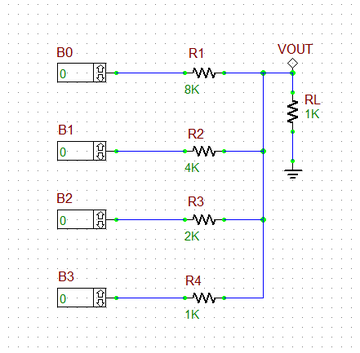The basic D/A converter circuit with a digital clock.

Note that the resistor R1 is twice the value of R2, and R2 is twice the value of R3, and so on. The four digital inputs B0, B1, B2 and B3 together form a 4-bit digital word. The corresponding voltages are then added at the load resistor RL. It can be shown that the output voltage is given by:

$V_o = \frac {R_L V_h}{ R + (2^N - 1) R_L } \left( S_{N-1} 2^{N-1} + S_{N-2} 2^{N-2} + ... + S_1 2^1 + S_0 2^0 \right)$

where vh is the high digital voltage, RL is the load resistor, R is the largest resistor value corresponding to the LSB input and Si represents the ith binary digit (S0 = LSB and SN-1 = MSB). For your circuit, N = 4, vh = +5V, RL = 1k, R = 8k, and you can write:

$V_o = \frac {5}{23} \left( 8S_3 + 4S_2 + 2S_1 + S_0 \right)$

When all four digital inputs are low, the output voltage is zero. For "0001", the output voltage is 217.4mV, and for "0010", the output voltage is 652.2mV. For the largest possible 4-bit binary number "1111", the output voltage is 3.261V. Run a Transient Test of the basic D/A converter with the start and stop time set to 0 and 10ms, respectively, with a Step Ceiling of 1μs. Set different combinations of the four binary bits and plot the output voltage. Some of these graphs are shown in the figures below, and their results agree with the above analytical calculations.The analog output voltage of the basic D/A converter when B3B2B1B0 = 0001.The analog output voltage of the basic D/A converter when B3B2B1B0 = 0011.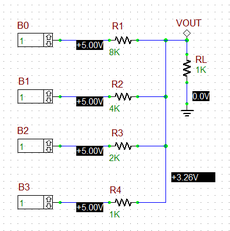The analog output voltage of the basic D/A converter when B3B2B1B0 = 1111.

## Building an R-2R Ladder D/A Converter

The following is a list of parts needed for this part of the tutorial lesson:

Part Name Part Type Part Value
B0 - B3 Digital Input 1-bit
A1 - A4 Generic AND Gate Defaults
CLK Digital Clock Period = 1m, Pulse Width = 500u
R1 - R4 Resistor 2k
R5 - R7 Resistor 1k
R8 - R9 Resistor 2k
R10 Resistor 1k
Vcc DC Bias Voltage Source 15V
Vee DC Bias Voltage Source -15V
X1 LM741 Op-Amp Defaults
VOUT Voltage Probe Marker N/A

The basic D/A converter of the previous section required a wide range of resistor values. The R-2R ladder D/A Converter shown in the figure below requires only two values R and 2R connected successively in a ladder configuration. This design also features a digital control circuit of this circuit. The digital inputs are transferred to the resistors only during the high state of the clock due to the AND gates. Set the period and pulse width of the digital clock CLK to 1ms and 500μs, respectively. An Op-Amp voltage follower has been added at the output through a coupling resistor R10 as a buffer. Place and connect all the parts and use an LM741 Op-Amp for the voltage follower stage.The R-2R Ladder D/A converter circuit with an Op-Amp.

Run a Transient Test of the ladder D/A converter with the following parameters:

 Start Time 0 10m 1u 1u Defaults

Setting different combinations of the four binary bits, you can verify the operation of this D/A converter and get very similar results as in the previous case. The graphs below show the results for the case of the 4-bit binary number "0001", "0011" and "1111".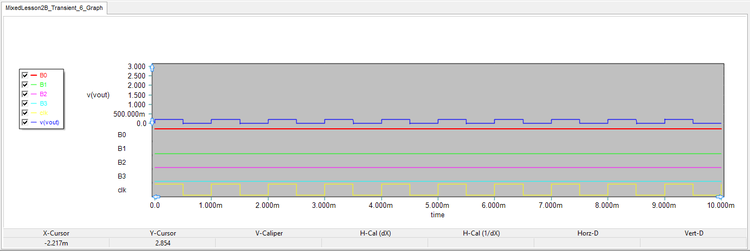The four digital inputs and the output analog voltage of the ladder D/A converter when B3B2B1B0 = 0001.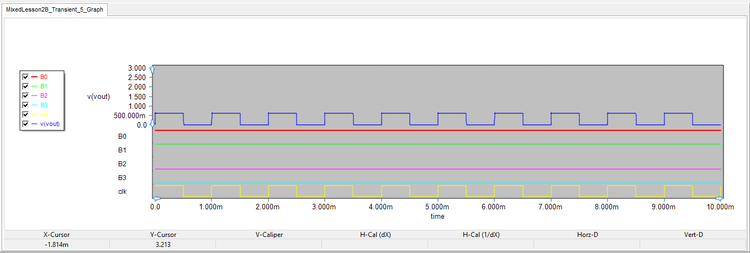The four digital inputs and the output analog voltage of the ladder D/A converter when B3B2B1B0 = 0011.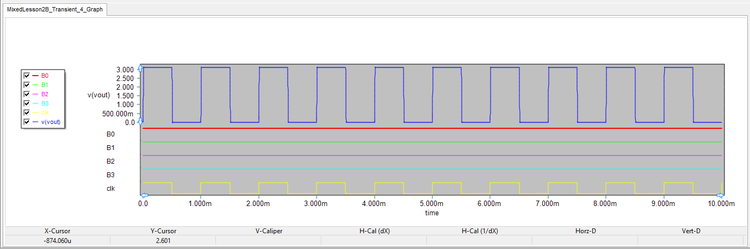The four digital inputs and the output analog voltage of the ladder D/A converter when B3B2B1B0 = 1111.